##### Physics I For Dummies, 3rd EditionIn physics, you can examine certain properties of molecules of an ideal gas as they zip around. For instance, you can calculate the average kinetic energy (and speed) of each molecule with a very simple equation: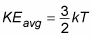where k is Boltzmann’s constant,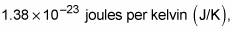and T is the temperature in kelvins. And because you can determine the mass of each molecule if you know which gas you’re dealing with, you can figure out the molecules’ speeds at various temperatures.

Imagine you’re at a picnic with friends on a beautiful spring day. You can’t see the air molecules whizzing around you, but you can predict their average speeds. You get out your calculator and thermometer. You measure the air temperature at about 28 degrees Celsius, or 301 kelvin. You know that for the molecules in the air, you can calculate their average kinetic energy withNow plug in the numbers: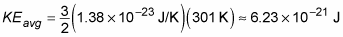The average molecule has a kinetic energy of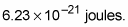The molecules are pretty small — what speed does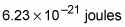correspond to? Well, you know that KE = (1/2)mv2 where m is the mass and v is the velocity. Therefore,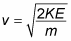Air is mostly nitrogen, and each nitrogen atom has a mass of about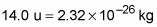(you can figure that one out yourself by finding the mass of a mole of nitrogen and dividing by the number of atoms in a mole, NA). In air, nitrogen molecules form molecules comprising two nitrogen atoms, so the mass of these molecules is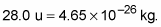You can plug in the numbers to get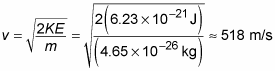Yow! What an image; huge numbers of the little guys crashing into you at 1,160 miles per hour! Good thing for you the molecules are so small. Imagine if each air molecule weighed a couple of pounds. Big problems.